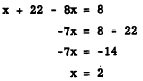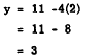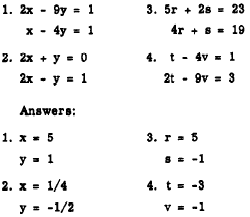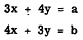Custom SearchSubstitution Method In some cases it is more convenient to use the substitution method of solving problems. In this method we solve one equation for one of the variables and substitute the value obtained into the other equation. This eliminates one of the variables, leaving an equation in one unknown. For example, find the solution of the following system: - .It is easy to solve for either y in the first equation or x in the second equation. Let us solve for y in the first equation. The result is y = 11 - 4x Since equals may be substituted for equals, we may substitute this value of y wherever y appears in the second equation. Thus, x + 2(11 - 4x) = 8 We now have one equation that is linear in x; that is, the equation contains only the variable x. Removing the parentheses and solving for x, we find thatTo get the corresponding value of y, we substitute x = 2 in y = 11 - 4x. The result isThus, the solution for the two original equations is x = 2 and y = 9.. Practice problems. Solve the following systems by the substitution method:Literal Coefficients Simultaneous equations with literal coefficients and literal constants may be solved for the value of the variables just as the other equations discussed in this chapter, with the exception that the solution will contain literal numbers. For example, find the solution of the system:We proceed as with any other simultaneous linear equation. Using the addition method, we may proceed as follows: To eliminate the y term we multiply the first equation by 3 and the second equation by -4. The equations then becomeTo eliminate x, we multiply the first equation by 4 and the second equation by -3. The equations then becomeWe may check in the same manner as that used for other equations, by substituting these values in the original equations.Integrated Publishing, Inc. - A (SDVOSB) Service Disabled Veteran Owned Small Business